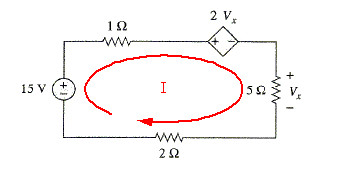# Circuits-dependent source

## Homework Statement

i attached the problem.
I am unsure how to solve this problem. I applied KVL and got 15-1I-2vx-vx-2I=0. Where I is the current in the circuit. From that equation I have 2 unknowns, so I obviously have to find I.

To find I I'd have to find Req, but I don't know how the resistors are arranged. the 2 ohm and 5ohm resistors are in series giving an equivalent R=7ohms. Is the 1ohm resistor in parallel with the combination R=7ohm?

#### Attachments

•Untitled.png
6.9 KB · Views: 379

gneill
Mentor
It's a series circuit -- all the components are connected in series.

It's a series circuit -- all the components are connected in series.

I don't get how it's a series circuit. Isn't the voltage dependent voltage source (2vx) separating the 1 ohm resistor from being in series with the others?

gneill
Mentor
I don't get how it's a series circuit. Isn't the voltage dependent voltage source (2vx) separating the 1 ohm resistor from being in series with the others?

No, the dependent source is just another component in the loop. Note how there is only one path for current to follow around the single loop. This is a series circuit.#### Attachments

No, the dependent source is just another component in the loop. Note how there is only one path for current to follow around the single loop. This is a series circuit.Oh I see thanks. Does that dependent source have an effect on calculating the current? For this problem I found Req=8ohms. Would current just be 15V/8ohms=I or do I have to take into account the dependent source?

gneill
Mentor
Oh I see thanks. Does that dependent source have an effect on calculating the current? For this problem I found Req=8ohms. Would current just be 15V/8ohms=I or do I have to take into account the dependent source?

The dependent source certainly has an effect on the current.

Start by writing the KVL equation for the loop.

I did and got 15-1I-2vx-vx-2I=0.
Would my other equation be V=IR, I=(15-2vx)/8

gneill
Mentor
I did and got 15-1I-2vx-vx-2I=0.
Would my other equation be V=IR, I=(15-2vx)/8

I suppose you could use that equation, but why not something simpler, like

Vx = I*5Ω ?

I suppose you could use that equation, but why not something simpler, like

Vx = I*5Ω ?

Yeah I just realized the Circuit said what the drop was over the 5 ohm resistor